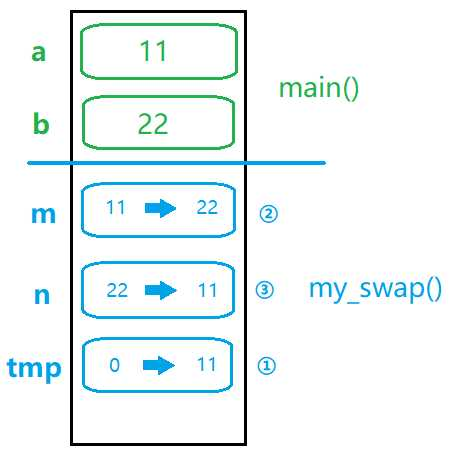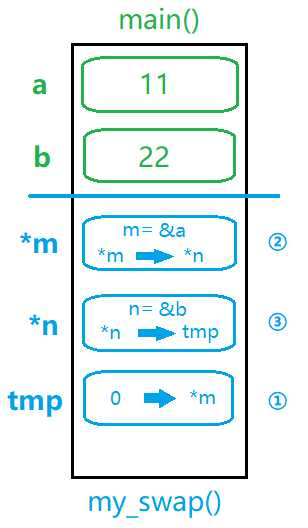# 参数中的值传递和地址传递

```void my_swap(int m,int n)

{
int tmp = 0;

tmp = m;m = n;n = tmp;

printf("m = %d,n = %d\n",m,n) ;

}

int main()

{
int a = 11,b = 22;

my_swap(a,b);

printf("a = %d,b = %d\n",a,b);
}``````void my_swap(int *m ,int *n)

{
int tmp = 0;

tmp = *m;//将m指向的变量的值赋值给tmp

*m = *n;//将n指向的变量的值给了m指向的变量

*n = tmp;//将tmp的值给了n指向的变量

}   ```(0)
(0)